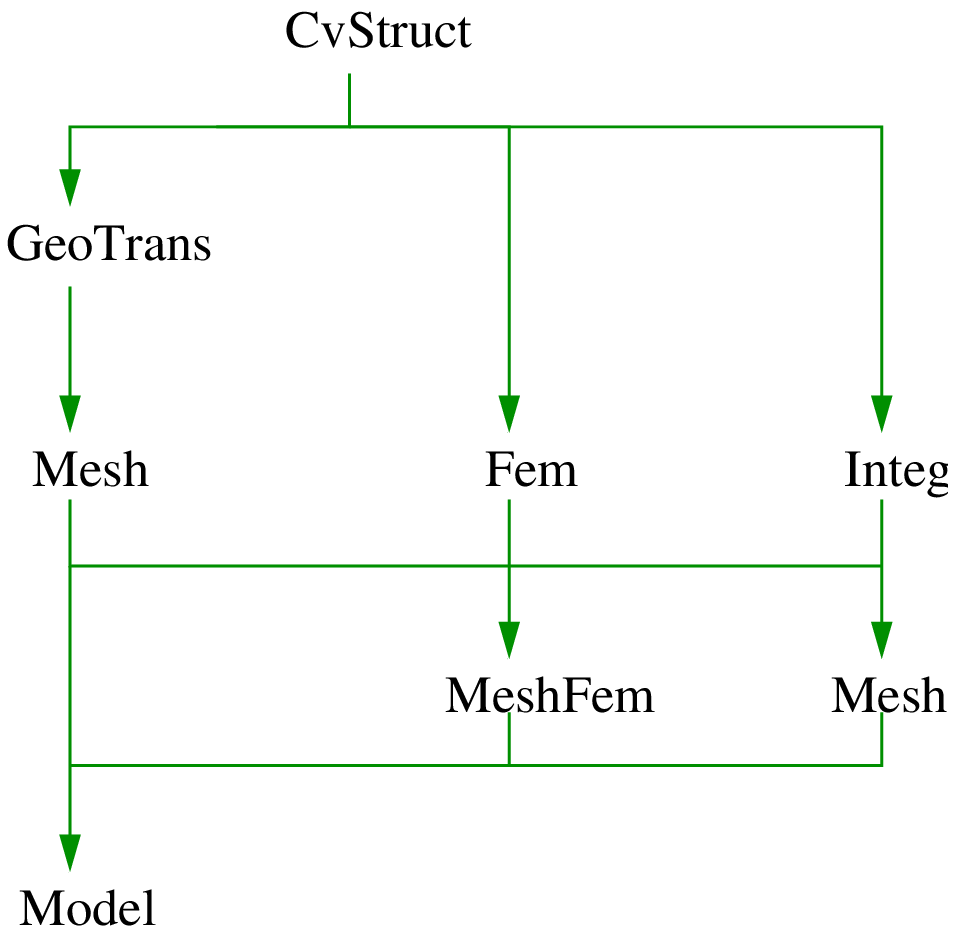# PythonGetFEM++ interface¶

## Introduction¶

GetFEM++ provides an interface to the Python scripting language. Python is a nice, cross-platform, and free language. With the addition of the numpy package, python provides a subset of Matlab functionalities (i.e. dense arrays). The VTK toolkit may provide visualization tools via its python interface (or via MayaVi), and data files for OpenDX may be exported. In this guide, nevertheless, to visualize the results, we will export to Gmsh post-processing format. The sparse matrix routines are provided by the getfem interface.

The python interface is available via a python module getfem.py. In order to use the interface you have to load it with:

```import getfem
m = getfem.Mesh('cartesian', range(0, 3), range(0,3))
```

or:

```from getfem import *
m = Mesh('cartesian', range(0, 3), range(0,3))
```

If the getfem.py (and the internal _getfem.so) module is not installed in a standard location for python, you may have to set the PYTHONPATH environment variable to its location. For example with:

```import sys
sys.path.append('.../getfem/getfem++/interface/src/python/')
```

## Parallel version¶

The python interface is the only one for the moment to interface the mpi based parallel version of Getfem. See MPI Parallelization of GetFEM++.

## Memory Management¶

A nice advantage over the Matlab interface is that you do not have to explicitly delete objects that are not used any more, this is done automagically. You can however inspect the content of the getfem workspace with the function getfem.memstats().

## Documentation¶

The getfem module is largely documented. This documentation has been extracted into the API reference. The getfem-matlab user guide may also be used, as 95% of its content translates quite directly into python (with the exception of the plotting functions, which are specific to matlab).

## PythonGetFEM++ organization¶

The general organization of the python-interface is the following:

• Each class from the matlab interface has a corresponding class in the python interface: the gfMesh class becomes the getfem.Mesh class in python, the gfSlice becomes the getfem.Slice etc.

• Each get and set method of the matlab interface has been translated into a method of the corresponding class in the python interface. For example:

```gf_mesh_get(m, 'outer faces');
gf_mesh_get(m, 'pts');
```

becomes:

```m.outer_faces();
m.pts();
```

Some methods have been renamed when there was ambiguity, for example gf_mesh_set(m, 'pts', P) is m.set_pts(P).

• The other getfem-matlab function have a very simple mapping to their python equivalent:

 gf_compute(mf,U,’foo’,...) getfem.compute_foo(mf,U) or getfem.compute(‘foo’,...) gf_asm(‘foobar’,...) getfem.asm_foobar(...) or getfem.asm(‘foobar’,...) gf_linsolve(‘gmres’,...) getfem.linsolve_gmres(...) or getfem.linsolve(‘gmres’,...)python-getfem interface main objects hierarchy.

class CvStruct(self, *args)

Descriptor for a convex structure objects, stores formal information convex structures (nb. of points, nb. of faces which are themselves convex structures)

class GeoTrans(self, *args)

Descriptor for geometric transformations objects (defines the shape/position of the convexes).

class Mesh(self, *args)

Descriptor for mesh structure (nodes, convexes, geometric transformations for each convex).

class Fem(self, fem_name)

Descriptor for FEM (Finite Element Method) objects (one per convex, can be PK, QK, HERMITE, etc...).

class Integ(self, *args)

Descriptor for Integration Method objects (exact, quadrature formulaldots). Although not linked directly to GeoTrans, an integration method is usually specific to a given convex structure.

class MeshFem(self, *args)

Descriptor for object linked to a mesh, where each convex has been assigned a FEM.

class MeshIm(self, *args)

Descriptor for object linked to a mesh, where each convex has been assigned an integration method.

class Model(self, *args)

Descriptor for model object, holds the global data, variables and description of a model. Evolution of model state and model brick object for 4.0 version of GetFEM++.

Preliminary

Examples